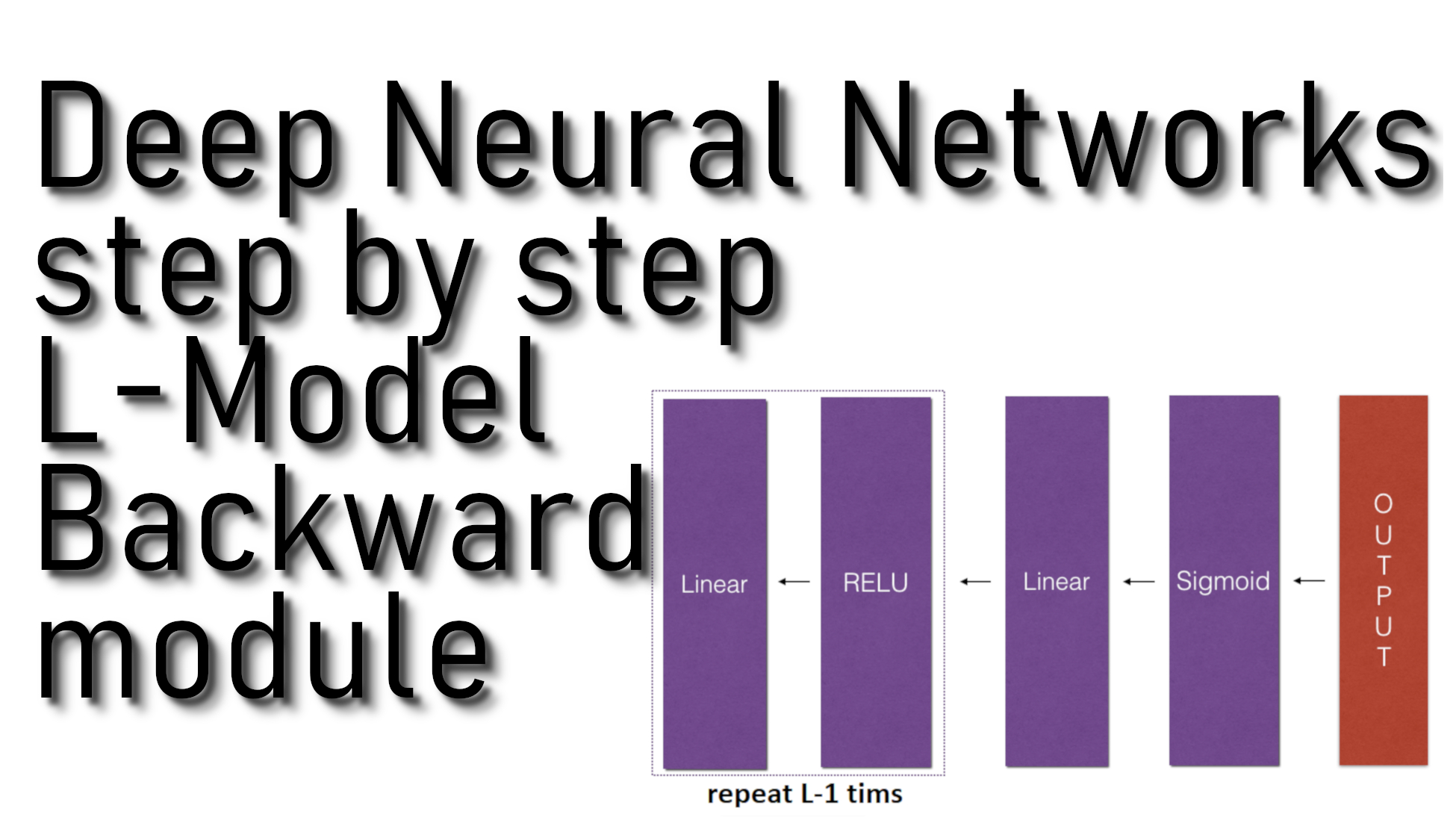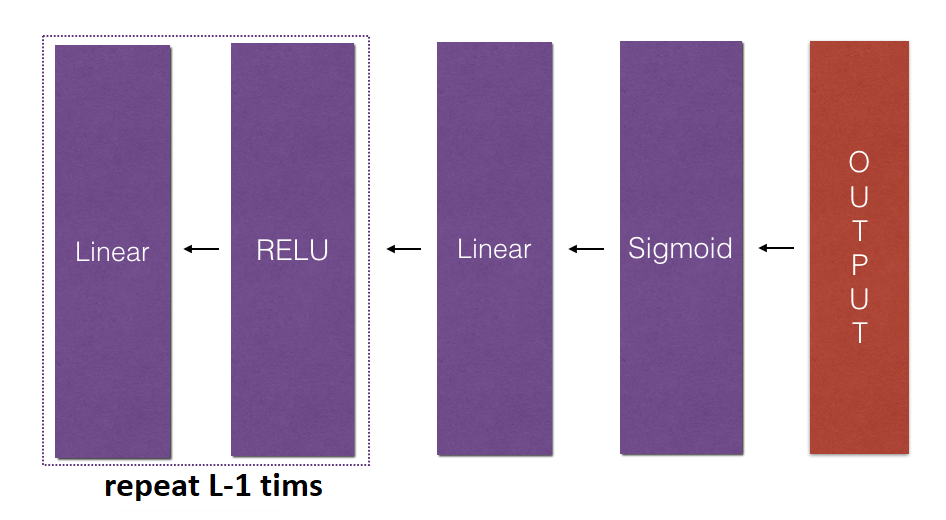# Deep Neural Networks Backward module

## In this part, we will implement the backward function for the whole network and we will also update the parameters of the model, using gradient descent

The Most Advanced Data Science Roadmaps You've Ever Seen! Comes with Thousands of Free Learning Resources and ChatGPT Integration! https://aigents.co/learn/roadmaps/intro

## L-Model Backward module:

In this part, we will implement the backward function for the whole network. Recall that when we implemented the L_model_forward function, we stored a cache containing a cache at each iteration (X, W, b, and z). In the backpropagation module, we will use those variables to compute the gradients. Therefore, in the L_model_forward function, we will iterate through all the hidden layers backward, starting from layer L. In each step, we will use the cached values for layer l to backpropagate through layer l. The figure below shows the backward pass.To backpropagate through this network, we know that the output is:

${A}^{\left[L\right]}=\sigma \left({Z}^{\left[L\right]}\right)$

Our code needs to compute:

$dAL=\frac{\partial L}{\partial {A}^{\left[L\right]}}$

To do so, we'll use this formula (derived using calculus which you don't need to remember):

# derivative of cost with respect to AL
dAL = - (np.divide(Y, AL) - np.divide(1 - Y, 1 - AL))

We can then use this post-activation gradient dAL to keep going backward. As seen in the figure above, we can now feed in dAL into the LINEAR->SIGMOID backward function we implemented (which will use the cached values stored by the L_model_forward function). After that, we will have to use a for loop to iterate through all the other layers using the LINEAR->RELU backward function. We should store each dA, dW, and db in the grads dictionary. To do so, we'll use this formula:

$grads\left["dW"+str\left(l\right)\right]=d{W}^{\left[l\right]}$

For example, for l=3 this would store dW[l] in grads["dW3"].

## Code for our linear_backward function:

Arguments:

AL - probability vector, the output of the forward propagation L_model_forward();
Y - true "label" vector (containing 0 if non-cat, 1 if cat);
caches - list of caches containing:
1. every cache of linear_activation_forward() with "relu" (it's caches[l], for l in range(L-1) i.e l = 0...L-2);
2. the cache of linear_activation_forward() with "sigmoid" (it's caches[L-1]).

Return:

def L_model_backward(AL, Y, caches):

# the number of layers
L = len(caches)
m = AL.shape

# after this line, Y is the same shape as AL
Y = Y.reshape(AL.shape)

# Initializing the backpropagation
dAL = - (np.divide(Y, AL) - np.divide(1 - Y, 1 - AL))

current_cache = caches[L-1]

# Loop from l=L-2 to l=0
for l in reversed(range(L-1)):
# lth layer: (RELU -> LINEAR) gradients.
# Inputs: "grads["dA" + str(l + 1)], current_cache".
# Outputs: "grads["dA" + str(l)] , grads["dW" + str(l + 1)] , grads["db" + str(l + 1)]

current_cache = caches[l]
dA_prev_temp, dW_temp, db_temp = linear_activation_backward(grads["dA"+str(l+1)], current_cache, "relu")
grads["dW" + str(l + 1)] = dW_temp
grads["db" + str(l + 1)] = db_temp

return grads

## Update Parameters module:

In this section, we will update the parameters of the model using gradient descent:

${W}^{\left[l\right]}={W}^{\left[l\right]}-\alpha d{W}^{\left[l\right]}\phantom{\rule{0ex}{0ex}}{b}^{\left[l\right]}={b}^{\left[l\right]}-\alpha d{b}^{\left[l\right]}$

here α is the learning rate. After computing the updated parameters, we'll store them in the parameters dictionary.

## Code for our update_parameters function:

Arguments:

parameters - python dictionary containing our parameters.

Return:

parameters - python dictionary containing our updated parameters:
parameters["W" + str(l)] = ...
parameters["b" + str(l)] = ...

def update_parameters(parameters, grads, learning_rate):
# number of layers in the neural network
L = len(parameters) // 2

# Update rule for each parameter
for l in range(L):
parameters["W" + str(l+1)] = parameters["W" + str(l+1)] - learning_rate*grads["dW" + str(l+1)]
parameters["b" + str(l+1)] = parameters["b" + str(l+1)] - learning_rate*grads["db" + str(l+1)]

return parameters

Conclusion:

Congrats on implementing all the functions required for building a deep neural network. It was a long tutorial, but from now on, it will only get better. We'll put all these together to build An L-layer neural network (deep) in the next part. In fact, we'll use these models to classify cat vs. dog images.